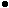1.1.11. The Hot Big Bang Model

On the basis of two observations, 1) the Universe is currently in a state of uniform expansion and 2) the Universe is filled with photons that come from background radiation at a temperature of 2.74 K, we can construct our generic cosmological model known as the Hot Big Bang model. This model is but a mere 30 years old so we should not expect it to be a complete description of what we observe and indeed it is not. What the model can explain well is the following:The Origin of the CMB: From its initially extremely hot and dense state, the CMB arises due to photon production from matter-anti-matter annihilation. Once the photon background is produced it simply cools with the expansion of the Universe, in the thermodynamic manner first specified by Gamow. The observed entropy of the Universe, S, which is measured by the ratio of baryons to photons is5 x 10-10. Essentially this means for every 5 billion anti-quarks, there was 5 billion +1 quarks. The residue of this tiny mass asymmetry has produced the observed stars and galaxies in the Universe.The Uniform Expansion of the Universe: By simple extrapolation to very small times, the currently observed expansion law, V = HD, must produce a situation where all the matter is concentrated into a small volume. At some point in this small volume, unknown physics initiated the expansion. Since the observed expansion today shows no preferred direction then the expansion became isotropic very early on.The Abundance of Light Elements: The Universe at expansion age of about one second was a hot and dense mixture of electrons, protons, neutrons, neutrinos and photons. The ratio of protons to neutrons at this time was unity as interactions with neutrinos mediated the neutron to proton and proton to neutron conversion. However, at expansion age of 2 seconds, the Universe had cooled to the point where it became transparent to neutrinos and this mediation was gone. Since free neutrons decay with 1/2 life of900 seconds, the proton-to-neutron (p / n)ratio began to increase. As the Universe continued to expand, it cooled to the point where some the nucleons could fuse into light elements such as Deuterium and Helium through the same series of fusion reactions that are presently occurring in our Sun. At the time of this hydrogen to helium fusion p / n was 7. Thus for every 14 protons there were 2 neutrons. Since the proton-proton chain ultimately binds two protons to two neutrons so that 4He is produced, then 12 protons plus 1 4He nucleus results. Since the mass of 1 4He is very nearly equal to the mass of 4 protons (Hydrogen atoms), then an initial p / n of 7 makes a definite prediction about the 4He baryonic mass fraction of the Universe. Since the total baryonic mass of the Universe is essentially the mass in Hydrogen plus the mass in Helium then the Helium mass fraction is: (Mass of Helium) / (Mass of Hydrogen + Helium) or, in units of the proton mass, (4) / (4+12) = 1/4. The observed abundances of Deuterium, 3He and 4He are all quite consistent with simple predictions based on the theoretical conditions of the Universe at time 1-2 seconds (see Kernin and Sarkar 1996).

These three observations really form the observation foundation of the Hot Big Bang model. A fourth observation, that the Universe is filled with galaxies that are arranged in a complex structure, can not easily be accounted for in this model. While the general idea that structure formation via gravitational instability should produce observable anisotropies in the CMB is consistent with our observations, the overall complexity of the observed distribution of galaxies is not well understood yet. We will examine this issue in great detail in later chapters.